Question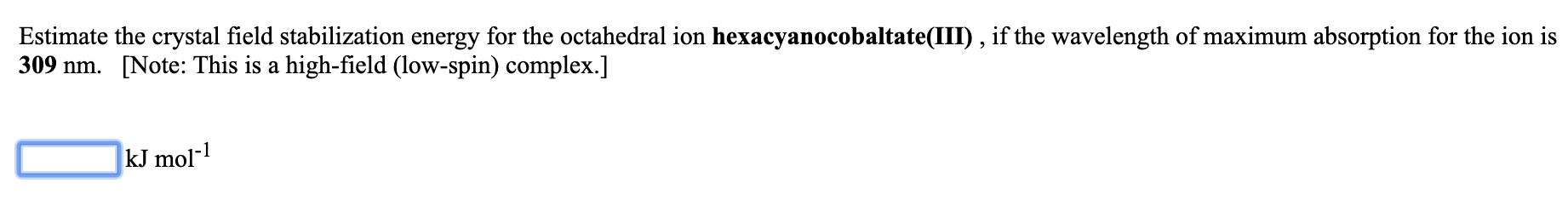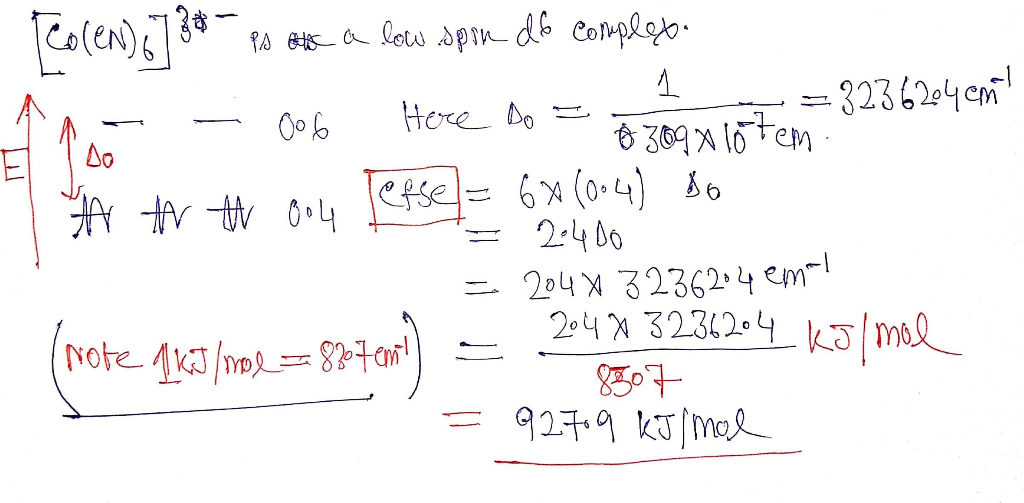#### Earn Coins

Coins can be redeemed for fabulous gifts.

Similar Homework Help Questions
• ### Estimate the crystal field stabilization energy for the octahedral ion hexacyanomanganate(III), if the wavelength of maximum...

Estimate the crystal field stabilization energy for the octahedral ion hexacyanomanganate(III), if the wavelength of maximum absorption for the ion is 600 nm. [Note: This is a high-field (low-spin) complex.] kJ mol-1

• ### Estimate the crystal field stabilization energy for the octahedral ion hexafluorocobaltate(III), if the wavelength of maximum...

Estimate the crystal field stabilization energy for the octahedral ion hexafluorocobaltate(III), if the wavelength of maximum absorption for the ion is 710 nm.   [Note: This is a weak-field (high-spin) complex.] _____ kJ mol-1

• ### 1) Estimate the crystal field stabilization energy for the octahedral ion hexaisothiocyanatotitanate(III), if the wavelength of...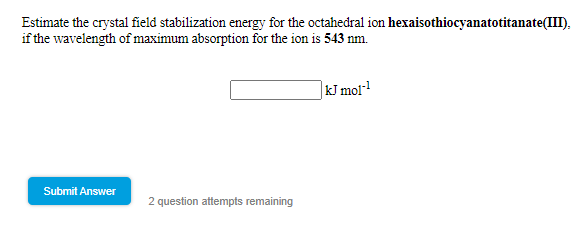1) Estimate the crystal field stabilization energy for the octahedral ion hexaisothiocyanatotitanate(III), if the wavelength of maximum absorption for the ion is 543 nm Estimate the crystal field stabilization energy for the octahedral ion hexaisothiocyanatotitanate(III). if the wavelength of maximum absorption for the ion is 543 nm. kJ moi? Submit Answer 2 question attempts remaining

• ### 620 nm Estimate the wavelength of maximum absorption for the octahedral ion trioxalatochromate(III) from the fact...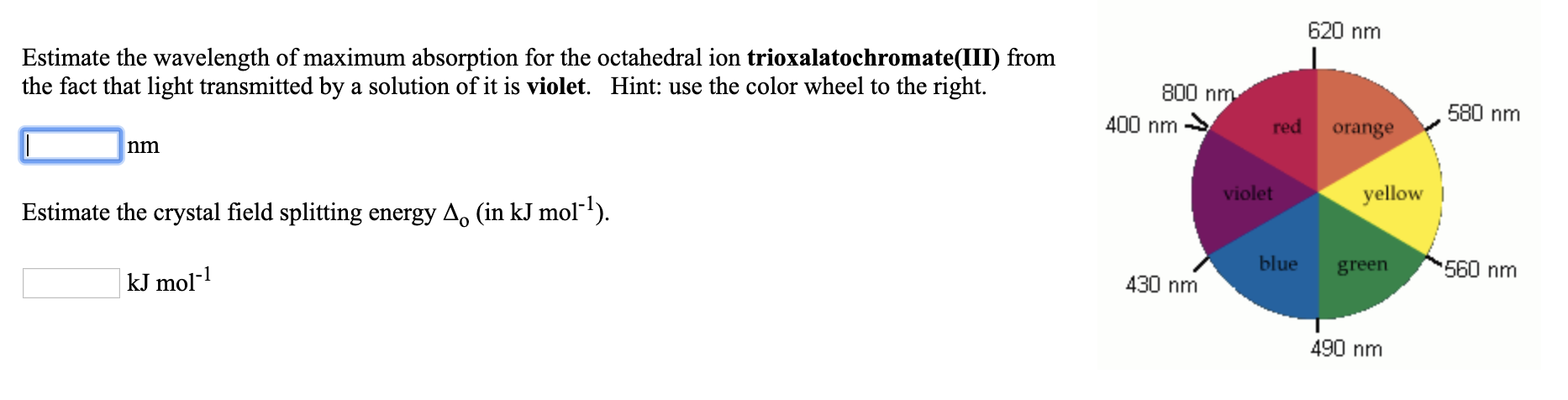620 nm Estimate the wavelength of maximum absorption for the octahedral ion trioxalatochromate(III) from the fact that light transmitted by a solution of it is violet. Hint: use the color wheel to the right. 800 nm 400 nm → red orange 580 nm violet yellow Estimate the crystal field splitting energy A. (in kJ mol-?). blue green kJ mol-1 560 nm 430 nm 490 nm

• ### Estimate the wavelength of maximum absorption for the octahedral ion hexaamminecobalt(lII) from right the fact that...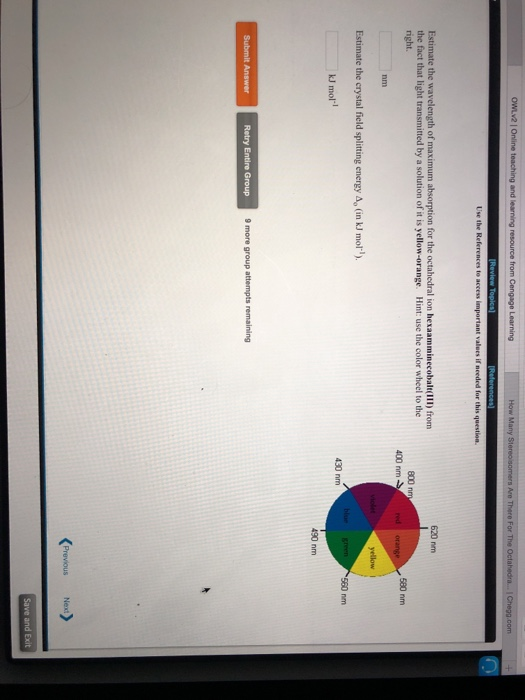Estimate the wavelength of maximum absorption for the octahedral ion hexaamminecobalt(lII) from right the fact that light transmitted by a solution of it is yellow-orange. Hint: use the color wheel to the 620 nm 800 nm 400 nm 580 nm nm Estimate the crystal field splitting energy Ap (in kJ mol) kJ mol-l 430 nm 490 nm Retry Entire Group 9 more group attempts remaining

• ### A ?1 octahedral complex is found to absorb visible light, with the absorption maximum occurring at 499 nm . Calculate the crystal-field splitting energy, Δ , in kJ/mol.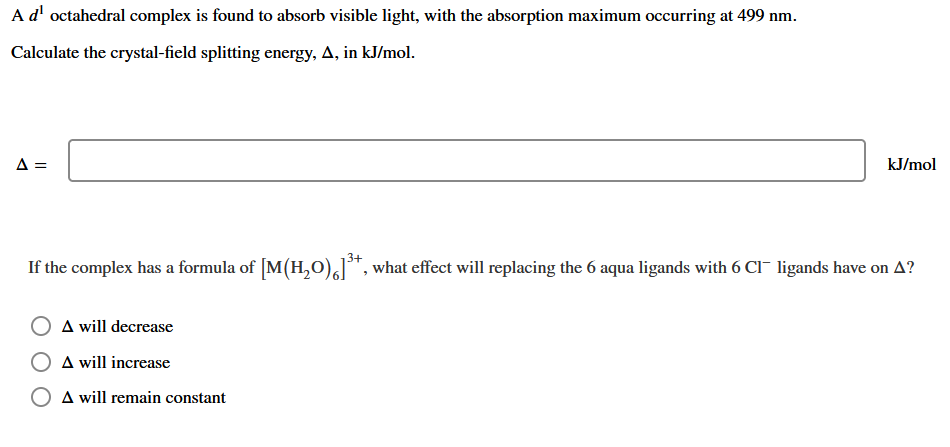A ?1 octahedral complex is found to absorb visible light, with the absorption maximum occurring at 499 nmCalculate the crystal-field splitting energy, Δin kJ/mol.

• ### The extent of crystal field splitting is often determined from spectra. Given the wavelength (7.) of...The extent of crystal field splitting is often determined from spectra. Given the wavelength (7.) of maximum absorption, 2. = 295 nm, find the crystal field splitting energy (A), in kJ/mol, for the complex ion (Rh(NH3).13+. kJ/mol

• ### 13. An octahedral transition metal complex with a d' electron configuration absorbs light of wavelength equal to 650 nm. a. what is the magnitude of the octahedral crystal field splitting par...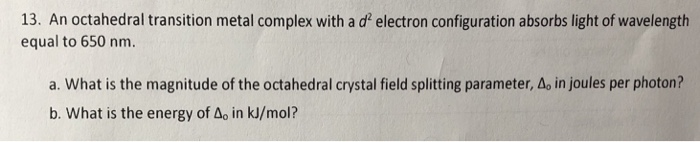13. An octahedral transition metal complex with a d' electron configuration absorbs light of wavelength equal to 650 nm. a. what is the magnitude of the octahedral crystal field splitting parameter, Δο in joules per photon? b. What is the energy of Ao in kJ/mol? 13. An octahedral transition metal complex with a d' electron configuration absorbs light of wavelength equal to 650 nm. a. what is the magnitude of the octahedral crystal field splitting parameter, Δο in joules per...

• ### Calculate the crystal field stabilization energy valiue for the complex Cr(NH)J*. The crystal field splitting energy...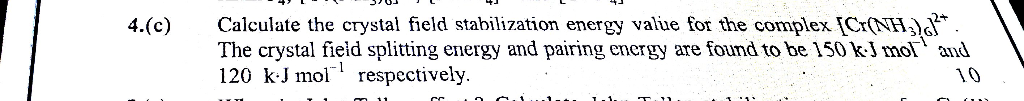Calculate the crystal field stabilization energy valiue for the complex Cr(NH)J*. The crystal field splitting energy and pairing energy are found to be 150 kJ mol and 120 kJ mol respectively. 4.(c) 10 cc. Calculate the crystal field stabilization energy valiue for the complex Cr(NH)J*. The crystal field splitting energy and pairing energy are found to be 150 kJ mol and 120 kJ mol respectively. 4.(c) 10 cc.

• ### Which dorbital configuration has the highest crystal field stabilization energy (CFSE) in a low spin octahedral...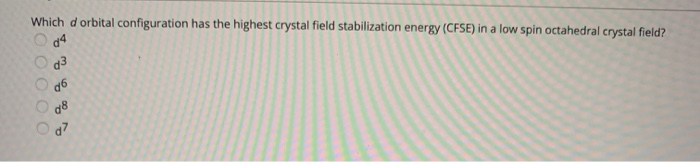Which dorbital configuration has the highest crystal field stabilization energy (CFSE) in a low spin octahedral crystal field? d4 d3 d6 ОООО g8 d? Predic whether the following complex is diamagnetic or paramagentic. 72+ paramagnetic need more information to answer this question diamagnetic The magnetic property of a transition metal complex can be predicted using crystal field theory. If we assume that the magnetic moment arises entirely from unpaired electron spins then the "spin only magnetic moment (us) is given...